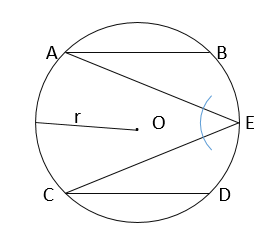# Geometry Is Fun! Huh!!the_belalReplay of MBSTU Lockdown...
Limits 1s, 512 MB

You are given a circle with center O and radius r. Two chords of the circle, AB and CD , are equal and parallel to each other. E is a point between B and D on the perimeter of the circle.You are given radius of the circle r and length of the chord AB. You have to calculate angle AEC.

## Input

First line will contain an integer T, the number of test cases (1<=T<=500) and the following T lines will contain exactly two integers r, AB ( 1<=r<=1000000000, AB<=2*r) where r denotes radius of the circle and AB denotes the length of the chord.

## Output

You have to print the output in the format “Case t: Y ” without quotes where t denotes test case number and Y denotes value of the angle AEC. Answer should be rounded to four places after the decimal point.

## Sample

InputOutput
3
5 8
8 11
156 192

Case 1: 36.8699
Case 2: 46.5675
Case 3: 52.0201


If you need you can use PI equals to acos(-1.0).GeometryuDebug

### Submit